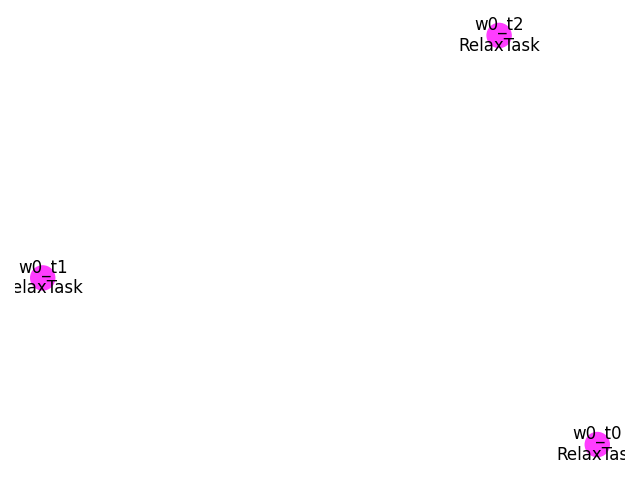# Gruneisen parameters with DFPT phonons and finite difference¶

This script compute the Grüneisen parameters (derivative of frequencies wrt Volume) using finite differences and the phonons obtained with the DFPT part of Abinit. The Anaddb input file needed to compute Grüneisen parameters will be generated in the outdata directory of the flow.

It is necessary to run three DFPT phonon calculations. One is calculated at the equilibrium volume and the remaining two are calculated at the slightly larger volume and smaller volume than the equilibrium volume. The unitcells at these volumes have to be fully relaxed under the constraint of each volume.Out:

/Users/gmatteo/git_repos/pymatgen/pymatgen/util/plotting.py:550: UserWarning: Matplotlib is currently using agg, which is a non-GUI backend, so cannot show the figure.
plt.show()


import sys
import os
import abipy.abilab as abilab
import abipy.data as abidata
from abipy import flowtk

def build_flow(options):
"""
Create a Flow for Grüneisen calculations:
Three relaxations at fixed volume followed by phonon calculation on a q-mesh.
"""
# Working directory (default is the name of the script with '.py' removed and "run_" replaced by "flow_")
if not options.workdir:
options.workdir = os.path.basename(sys.argv).replace(".py", "").replace("run_", "flow_")

flow = flowtk.Flow(workdir=options.workdir)

# This function constructs the input files for the phonon calculation:
# GS input + the input files for the phonon calculation.
pseudos = abidata.pseudos("14si.pspnc")
structure = abidata.structure_from_ucell("Si")

gs_inp = abilab.AbinitInput(structure, pseudos=pseudos)

# Global variables used both for the GS and the DFPT run.
gs_inp.set_vars(
nband=4,
ecut=2.0,
ngkpt=[4, 4, 4],
nshiftk=4,
shiftk=[0.0, 0.0, 0.5,   # This gives the usual fcc Monkhorst-Pack grid
0.0, 0.5, 0.0,
0.5, 0.0, 0.0,
0.5, 0.5, 0.5],
diemac=12.0,
iomode=3,
tolvrs=1.0e-18,
)

from abipy.flowtk.gruneisen import GruneisenWork
voldelta = gs_inp.structure.volume * 0.02

# k-mesh and q-mesh must be commensurate.
work = GruneisenWork.from_gs_input(gs_inp, voldelta, ngqpt=[2, 2, 2], with_becs=False)
flow.register_work(work)

return flow

# This block generates the thumbnails in the AbiPy gallery.
# You can safely REMOVE this part if you are using this script for production runs.
__name__ = None
import tempfile
options = flowtk.build_flow_main_parser().parse_args(["-w", tempfile.mkdtemp()])
build_flow(options).plot_networkx(tight_layout=True)

@flowtk.flow_main
def main(options):
"""
This is our main function that will be invoked by the script.
flow_main is a decorator implementing the command line interface.
Command line args are stored in options.
"""
return build_flow(options)

if __name__ == "__main__":
sys.exit(main())


Total running time of the script: ( 0 minutes 0.567 seconds)

Gallery generated by Sphinx-Gallery